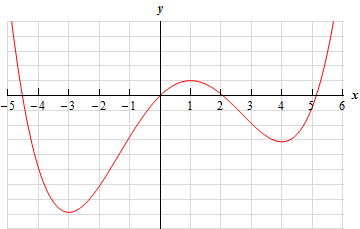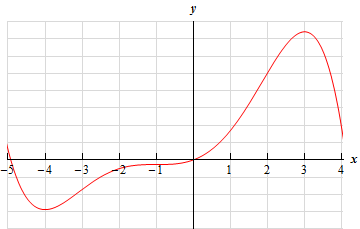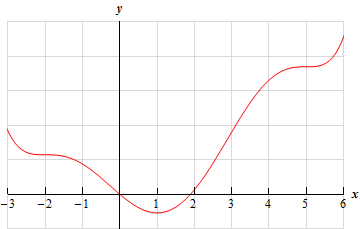Paul's Online Notes
Home / Calculus I / Applications of Derivatives / Critical Points
Show Mobile Notice Show All Notes Hide All Notes
Mobile Notice
You appear to be on a device with a "narrow" screen width (i.e. you are probably on a mobile phone). Due to the nature of the mathematics on this site it is best views in landscape mode. If your device is not in landscape mode many of the equations will run off the side of your device (should be able to scroll to see them) and some of the menu items will be cut off due to the narrow screen width.
Assignment Problems Notice
Please do not email me to get solutions and/or answers to these problems. I will not give them out under any circumstances nor will I respond to any requests to do so. The intent of these problems is for instructors to use them for assignments and having solutions/answers easily available defeats that purpose.

If you are looking for some problems with solutions you can find some by clicking on the "Practice Problems" link above.

### Section 4.2 : Critical Points

For problems 1 - 43 determine the critical points of each of the following functions. Note that a couple of the problems involve equations that may not be easily solved by hand and as such may require some computational aids. These are marked are noted below.

1. $$R\left( x \right) = 8{x^3} - 18{x^2} - 240x + 2$$
2. $$f\left( z \right) = 2{z^4} - 16{z^3} + 20{z^2} - 7$$
3. $$\displaystyle g\left( z \right) = 8 - 12{z^5} - 25{z^6} + \frac{90}{7}{z^7}$$
4. $$g\left( t \right) = 3{t^4} - 20{t^3} - 132{t^2} + 672t - 4$$
Note : Depending upon your factoring skills this may require some computational aids.
5. $$\displaystyle h\left( x \right) = 10{x^2} - 15{x^3} + \frac{15}{2}{x^4} - {x^5}$$
Note : Depending upon your factoring skills this may require some computational aids.
6. $$P\left( w \right) = {w^3} - 4{w^2} - 7w - 1$$
7. $$A\left( t \right) = 7{t^3} - 3{t^2} + t - 15$$
8. $$a\left( t \right) = 4 - 2{t^2} - 6{t^3} - 3{t^4}$$
9. $$f\left( x \right) = 3{x^4} - 20{x^3} + 6{x^2} + 120x + 5$$
Note : Depending upon your factoring skills this may require some computational aids.
10. $$h\left( v \right) = {v^5} + {v^4} + 10{v^3} - 15$$
11. $$g\left( z \right) = {\left( {z - 3} \right)^5}{\left( {2z + 1} \right)^4}$$
12. $$R\left( q \right) = {\left( {q + 2} \right)^4}{\left( {{q^2} - 8} \right)^2}$$
13. $$f\left( t \right) = {\left( {t - 2} \right)^3}{\left( {{t^2} + 1} \right)^2}$$
14. $$\displaystyle f\left( w \right) = \frac{{{w^2} + 2w + 1}}{{3w - 5}}$$
15. $$\displaystyle h\left( t \right) = \frac{{3 - 4t}}{{{t^2} + 1}}$$
16. $$\displaystyle R\left( y \right) = \frac{{{y^2} - y}}{{{y^2} + 3y + 8}}$$
17. $$Y\left( x \right) = \sqrt{{x - 7}}$$
18. $$f\left( t \right) = {\left( {{t^3} - 25t} \right)^{\frac{2}{3}}}$$
19. $$h\left( x \right) = \sqrt{x}\,\,{\left( {2x + 8} \right)^2}$$
20. $$Q\left( w \right) = \left( {6 - {w^2}} \right)\,\,\,\sqrt{{{w^2} - 4}}$$
21. $$\displaystyle Q\left( t \right) = 7\sin \left( \frac{t}{4} \right) - 2$$
22. $$g\left( x \right) = 3\cos \left( {2x} \right) - 5x$$
23. $$f\left( x \right) = 7\cos \left( x \right) + 2x$$
24. $$h\left( t \right) = 6\sin \left( {2t} \right) + 12t$$
25. $$\displaystyle w\left( z \right) = {\cos ^3}\left( \frac{z}{5} \right)$$
26. $$U\left( z \right) = \tan \left( z \right) - 4z$$
27. $$h\left( x \right) = x\cos \left( x \right) - \sin \left( x \right)$$
28. $$h\left( x \right) = 2\cos \left( x \right) - \cos \left( {2x} \right)$$
29. $$f\left( w \right) = {\cos ^2}\left( w \right) - {\cos ^4}\left( w \right)$$
30. $$F\left( w \right) = {{\bf{e}}^{14w + 3}}$$
31. $$g\left( z \right) = {z^2}{{\bf{e}}^{1 - z}}$$
32. $$A\left( x \right) = \left( {3 - 2x} \right){{\bf{e}}^{{x^{\,2}}}}$$
33. $$P\left( t \right) = \left( {6t + 1} \right){{\bf{e}}^{8t - {t^{\,2}}}}$$
34. $$f\left( x \right) = {{\bf{e}}^{3 + {x^{\,2}}}} - {{\bf{e}}^{2{x^{\,2}} - 4}}$$
35. $$f\left( z \right) = {{\bf{e}}^{{z^{\,2}} - 4z}} + {{\bf{e}}^{8z - 2{z^2}}}$$
36. $$h\left( y \right) = {{\bf{e}}^{6{y^{\,3}} - 8{y^{\,2}}}}$$
37. $$g\left( t \right) = {{\bf{e}}^{2{t^{\,3}} + 4{t^{\,2}} - t}}$$
38. $$Z\left( t \right) = \ln \left( {{t^2} + t + 3} \right)$$
39. $$G\left( r \right) = r - \ln \left( {{r^2} + 1} \right)$$
40. $$A\left( z \right) = 2 - 6z + \ln \left( {8z + 1} \right)$$
41. $$f\left( x \right) = x - 4\ln \left( {{x^2} + x + 2} \right)$$
42. $$g\left( x \right) = \ln \left( {4x + 2} \right) - \ln \left( {x + 4} \right)$$
43. $$h\left( t \right) = \ln \left( {{t^2} - t + 1} \right) + \ln \left( {4 - t} \right)$$
44. The graph of some function, $$f\left( x \right)$$, is shown. Based on the graph, estimate the location of all the critical points of the function.45. The graph of some function, $$f\left( x \right)$$, is shown. Based on the graph, estimate the location of all the critical points of the function.46. The graph of some function, $$f\left( x \right)$$, is shown. Based on the graph, estimate the location of all the critical points of the function.